Reach Us+44-7482-875032
Method of Forecasting an Oil Spreading on Water by Means of Neural Networks | OMICS International
Journal of Applied & Computational Mathematics
All submissions of the EM system will be redirected to Online Manuscript Submission System. Authors are requested to submit articles directly to Online Manuscript Submission System of respective journal.

# Method of Forecasting an Oil Spreading on Water by Means of Neural Networks

Ghasemi Habashi YA1, Gasimov BM2, Efendiyeva HC1 and Mutallimov MM1*

1Institute of Applied Mathematics of Baku State University, Z. Khalilov Street, 23, AZ1148 Baku City, Azerbaijan

2Azerbaijan State University of Economics, Baku, Istiqlaliyyat str., 6, AZ1001, Baku City, Azerbaijan

*Corresponding Author:
Mutallimov MM
Institute of Applied Mathematics of Baku State University
Z. Khalilov Street, 23, AZ1148 Baku City, Azerbaijan
Tel: 994125391595
E-mail: [email protected]

Received Date: July 14, 2014; Accepted Date: October 28, 2014; Published Date: November 07,2014

Citation: Ghasemi Habashi YA, Gasimov BM, Efendiyeva HC, Mutallimov MM (2014) Method of Forecasting an Oil Spreading on Water by Means of Neural Networks. J Appl Computat Math 3:189. doi: 10.4172/2168-9679.1000189

Copyright: © 2014 Ghasemi Habashi YA, et al. This is an open-access article distributed under the terms of the Creative Commons Attribution License, which permits unrestricted use, distribution, and reproduction in any medium, provided the original author and source are credited.

Visit for more related articles at Journal of Applied & Computational Mathematics

#### Keywords

Oil; Sea; Neural; Networks

#### Introduction

In a wide variety of disciplines it is of great practical importance to measure, to describe and compare the shapes of objects. In general terms, the shape of an object, data set, or image can be defined as the total of all information that is invariant under translation, rotation and isotropic rescaling. The field of shape analysis involves hence methods for the study of the shape of objects where location, rotation and scale can be removed. The two or more dimensional objects are summarized according to key points called landmarks. This approach provides an objective methodology for classification whereas even today in many applications the decision for classifying according to the appearance seems at most intuitive.

Statistical shape analysis is concerned with methodology for analyzing shapes in the presence of randomness. It is a mathematical procedure to get the information of two or more dimensional objects with a possible correction of size and position of the object. So objects with different size and/or position can be compared with each other and classified. To get the shape of an object without information about position and size, centralization and standardization procedures are used in some metric space.

Interest in shape analysis began in 1977. Kendall published  a note in which he introduced a new representation of shapes as elements of complex projective spaces. Mardia on the other hand investigated the distribution of the shapes of triangles generated by certain point processes, and in particular considered whether towns in a plain are spread regularly with equal distances between close towns.

The full details of this elegant theory which contains interesting areas of research for both probabilists and statisticians where published by Kendall and Bookstein . The details of the theory and further developments can be found in the textbooks [1,2].

#### Neural Network

Neural networks have been developed originally in order to understand the cognitive processes. Nowadays there are a lot of applications of neural networks as a mathematical method in quite different disciplines.

The term “neural networks” points to the model of a nerve cell, the neuron, and the cognitive processes carried and driven by the network of interacting neurons. A neuron perceives chemical and physical excitement from the environment by its dendrites. The neuron is processing this incoming data and sending the information to other neurons via axon and synapses.

McCulloch and Pitts implemented the biological processes of a nerve cell for the first time in the mathematical way. Nerve cells have to access and process incoming data in order to evaluate target information. Therefore the corresponding neural networks are called supervised neural networks. An unsupervised neural network has no target and is similar to a cluster algorithm.

The data consists of n variables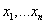on binary scale. For data processing, the i’th variable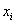is weighted with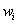Normalized with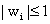1the multiplication ofwithdetermines the relevance offor a target . The value yreflects the correlation between the input variable and the target, the sign indicating the direction of the influence of the input variable on the target. Weighting the input variables for a target variables is similar to discriminant analysis .

The critical quantity for the neuron is the weighted sum of input variables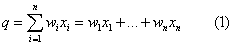For the target y with binary scale, a threshold S is needed. Crossing the threshold yields 1 and falling below the threshold yields 0. Hence the activation function F can be written as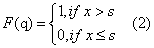In comparison to discriminant analysis, for neural networks the threshold S has to be assigned, depending on properties of the target; it cannot be derived from the data in a straightforward manner. Neural networks usually include no assumption about the data. Rather they are a numerical method .With the input of the activation function, we obtain y=F(q) as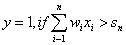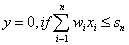Multi-layer Perceptrons

In general a given target may be reached only up to a certain error. Given a certain measure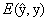for the distance between the given target state y and the state f computed by the neural network, learning of the neural network corresponds to minimization of.The following training algorithm is inspired by Rumelhart, Hinton and Williams. The total error measure over all states of a given layer is defined as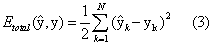Different kind of error measurements suited for the application can be applied. The upper error measurement will be used below to reset the weight in each layer of the neural network.

For simplicity, we consider now a 2-layer perceptron network, which also will be sufficient bellow for our purpose of calibrating the stochastic process.

The processed state The processed state ˆy of the neural network is computed by the following steps. First the critical parameter for the first layer is computed from n weighted input values as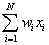we consider a hidden output layer with m neurons. For n let gj be the activation function of the j-th neuron of the hidden layer with an activation value of hj given as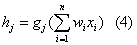Usually for all neurons of a given layer common activation function g={g1,….gm}, e.g. a sigmoid function, is used. Alternatively for simulating cyclical processes trigonometric function can be applied. This would be the case, if we assume that the same input value in combination with the time point has to be interpreted contrary. Next, the output of the previous (hidden) layer becomes the input of the next layer, and the activation proceeds analogously to the previous layer.

Let f be the activation function of the pre-final (here the second) output layer. Then the pre-final critical value is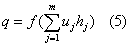Finally, the pre-final critical value q is interpreted by a final activation function F yielding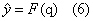As a final state value computed from the neural network with the given weight of the input variables from input and hidden layers.

Now the neural network performs a training step by modifying the weight of all input layers. The learning mechanism the weights are determined by the target distance measure (3) he weights of both layers are changed according to the steepest descent, i.e.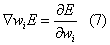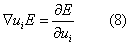With a learning rate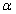which should be adapted to the data, the weights are changed as follows: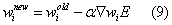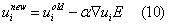#### Method of Solution

The necessary number of iterations depends on the requirements posed by the data, the user, and the discipline .

Instead of the error function, we are using the variance. We try to find an optimal variance for differentiating our groups.

Spreading of the oil spot in the sea and enlarge it, is harmful for sea animals and natural Geographic, to this reason and according to research it's very necessary to find out the size of spreading and can intercept or arrest it (Figures 1 and 2) .

The weather condition, gale energy, sun refulgence, water density and water clarity is very important parameters in oil spreading, but we want to solve the problem by neural networks without these problems.

We have so many methods to estimating the future of some things or process in different science, but also we know that the results of each method is different with another method and all of the results not really correct, results are closer to target.

Same we show in Figures 1 and 2, in our method first we determined 200 different points in outside circumference of oil spot, that these points are very close together, then get the all longitudes and latitudes of these points, and register these. We register new longitudes and latitudes of those points at each 6 hours on 5 sequential days, after 5 days we have new 20 longitudes and 20 latitudes for each point, then we input continual all 5 days data to the our neural network (Figure 3).

#### Results

Neural network features

1. non linear modeling capability

2. generic modeling capability

3. robustness to noisy data

4. ability for dynamic learning

5. requires availability of high density of data

Neural network modeling shows excellent promises for local forecasting of water levels, computationally and financially inexpensive method.

The quality of the wind forecasts will likely be the limiting factor for the accuracy of the water level forecasts (Figure 4).

First use historical time series of previous water levels, winds, barometric pressure as input then train neural network to associate changes in inputs and future water level changes after that make water level forecasts using a static neural network model (Figure 5).

Neural network modeling started in the 60's then key innovatation in the late 80's:backpropagation learning algorithms after that number of applications has grown rapidly in the 90's especially financial applications and then growing number of publications presenting environmentals (Figures 6 and 7).

#### Conclusion

In our learning algorithm we use previous data to estimate next data (like Fibonacci method). Now a day we begin to input all of data (5 day’s data) to network and according to our learning algorithm, this network give us the new longitudes and latitudes of those points on 6’th day (output) .

The points longitudes and latitudes on 6’th day, show us the estimating result of oil spot spreading (enlargement size) in the sea, that we can find it by means of neural network without using any human or other way.

But the best result of this network is, find out the estimating result of enlargement size on 9'th day without calculating results of 6'th, 7'th and 8'th days.

Note that using the results of [8,9] we can apply this technique to other practical problems.

#### References

Select your language of interest to view the total content in your interested language

### Article Usage

• Total views: 11811
• [From(publication date):
December-2014 - Aug 20, 2019]
• Breakdown by view type
• HTML page views : 8044
• PDF downloads : 3767

## Post your commentCan't read the image? click here to refresh
###### Peer Reviewed Journals

Make the best use of Scientific Research and information from our 700 + peer reviewed, Open Access Journals

International Conferences 2019-20

Meet Inspiring Speakers and Experts at our 3000+ Global Annual Meetings

Top